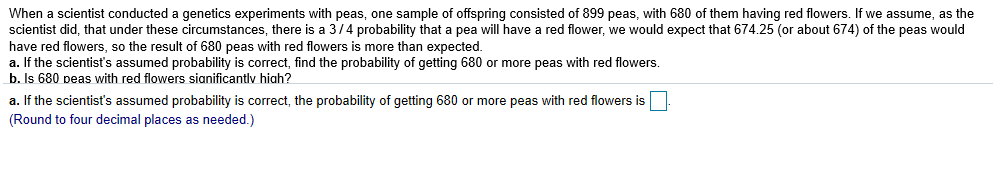When a scientist conducted a genetics experiments with peas, one sample of offspring consisted of 899 peas, with 680 of them having red flowers. If we assume, as thescientist did, that under these circumstances, there is a 3/4 probability that a pea will have a red flower, we would expect that 674.25 (or about 674) of the peas wouldhave red flowers, so the result of 680 peas with red flowers is more than expected.a. If the scientist's assumed probability is correct, find the probability of getting 680 or more peas with red flowers.b. Is 680 peas with red flowers sianificantlv hiah?a. If the scientist's assumed probability is correct, the probability of getting 680 or more peas with red flowers is(Round to four decimal places as needed.)

Questionhelp_outlineImage TranscriptioncloseWhen a scientist conducted a genetics experiments with peas, one sample of offspring consisted of 899 peas, with 680 of them having red flowers. If we assume, as the scientist did, that under these circumstances, there is a 3/4 probability that a pea will have a red flower, we would expect that 674.25 (or about 674) of the peas would have red flowers, so the result of 680 peas with red flowers is more than expected. a. If the scientist's assumed probability is correct, find the probability of getting 680 or more peas with red flowers. b. Is 680 peas with red flowers sianificantlv hiah? a. If the scientist's assumed probability is correct, the probability of getting 680 or more peas with red flowers is (Round to four decimal places as needed.) fullscreen
Step 1

Let X be the number of red flowers in the sample of offspring of 899 peas.

The total number of spring is 899.

The scientist has assumed that the probability of getting a pea that have red flower is 0.75.

That is the success event is getting a pea that have red flower and failure event is not getting a pea that have red flower.

The assumptions for binomial distribution:

• The number of trials is fixed.
• There are two outcomes success and failure with success probability p.
• Each of the trials will be independent.

Here, all the three assumptions are satisfied, therefore it can be said that X follows binomial distribution with n = 899, p = 0.75 (according to scientist’s assumption).

Step 2

The pmf of binomial distribution is,

Step 3

a.

Assuming the scientist’s assumed probability is correct, the probabi...

Want to see the full answer?

See Solution

Want to see this answer and more?

Our solutions are written by experts, many with advanced degrees, and available 24/7

See Solution
Tagged in

Other